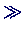## Basics: Vectors and Matrices

• Matlab is an interactive system for numerical calculations and visualization. It's basic element is the twodimensional array of floating point numbers, the matrix:A=[1 2 3; -1 0 2; 4 5 6]

A =

1     2     3
-1     0     2
4     5     6

• Vectors are just arrays with dimension 1xn (row vectors):B = [1 3 5]

B =

1     3     5

• or - using the matrix transposition -column vectors:B'

ans =

1
3
5

• We can now easily solve the system of linear equations A*X = B', using Matlab's "division" operator:X = A\ B'

X =

6.3333
-9.6667
4.6667

• and test the result by applying A to X:A*X

ans =

1.0000
3.0000
5.0000

• Larger systems are solved as easily, e.g. the following with random coefficientsA=rand(1000);B=rand(1000,1);X = A\ B;

• takes half a minute to solve on a (not too old) PC. We check the result by looking at the largest error:error = max(abs(A*X-B))

error =

1.9213e-012Peter Junglas 8.3.2000